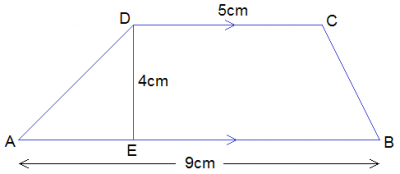### Mathematics Past Questions

4296

determine the maximum value of y=3x$$^2$$ + 5x - 3

• A. 6
• B. 0
• C. 2
• D. No correct option
JAMB 2021
4297A trapezium has two parallel sides of lengths 5cm and 9cm. If the area is 91cm$$^2$$, find the distance between the parallel sides

• A. 13 cm
• B. 4 cm
• C. 6 cm
• D. 7 cm
JAMB 2021
4298

find the value of p if the line which passes through (-1, -p) and (-2,2) is parallel to the line 2y+8x-17=0?

• A. $$\frac{-2}{7}$$
• B. $$\frac{7}{6}$$
• C. $$\frac{-6}{7}$$
• D. 2
JAMB 2021
4299

The ratio of the length of two similar rectangular blocks is 2 : 3. If the volume of the larger block is 351cm$$^3$$, then the volume of the other block is?

• A. 234.00 cm3
• B. 526.50 cm3
• C. 166.00 cm3
• D. 687cm3
JAMB 2021
4300

Find the derivative of the function y = 2x$$^2$$(2x - 1) at the point x = -1?

• A. 18
• B. 16
• C. -4
• D. -6
JAMB 2021